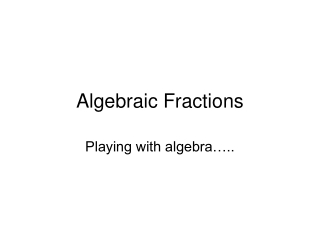# Algebraic Fractions - PowerPoint PPT PresentationDownload PresentationAlgebraic Fractions

Algebraic FractionsDownload Presentation## Algebraic Fractions

- - - - - - - - - - - - - - - - - - - - - - - - - - - E N D - - - - - - - - - - - - - - - - - - - - - - - - - - -
##### Presentation Transcript

1. Algebraic Fractions Playing with algebra…..

2. Starter • True false cards

3. Walt… • Simplify algebraic expressions • Add, subtract, multiply divide algebraic expressions. • Solve equations involving algebraic expressions.

4. http://www.mathsnet.net/asa2/2004/c31factor.html

5. http://www.mathsnet.net/asa2/2004/c31factor2.html